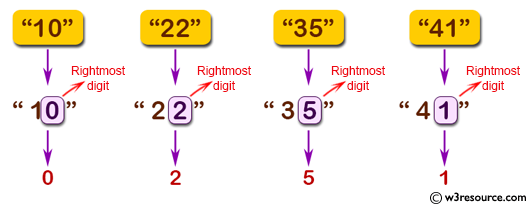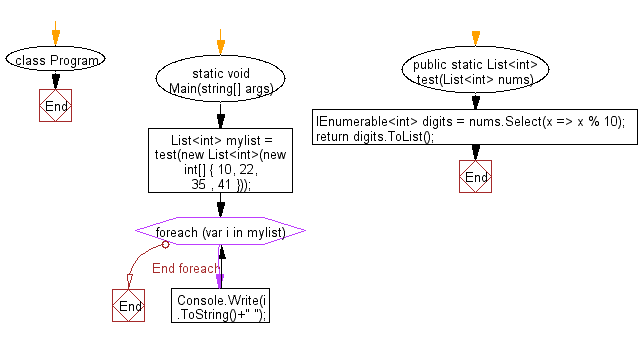﻿ C# Sharp exercises: Create a new list of the rightmost digits from a given list of positive integers - w3resource# C# Sharp Basic Algorithm Exercises: Create a new list of the rightmost digits from a given list of positive integers

## C# Sharp Basic Algorithm: Exercise-146 with Solution

Write a C# Sharp program to create a new list of the rightmost digits from a given list of positive integers.

Pictorial Presentation:Sample Solution:

C# Sharp Code:

``````using System;
using System.Collections.Gen eric;
using System.Linq;
namespace exercises
{
class Program
{
static void Main(string[] args)
{
List<int> mylist = test(new List<int>(new int[] { 10, 22, 35 , 41 }));
foreach(var i in mylist)
{
Console.Write(i.ToString()+" ");
}
}
public static List<int> test(List<int> nums)
{
IEnumerable<int> digits = nums.Select(x => x % 10);
return digits.ToList();
}
}
}
```
```

Sample Output:

`0 2 5 1`

Flowchart:C# Sharp Code Editor:

Improve this sample solution and post your code through Disqus

What is the difficulty level of this exercise?

﻿